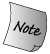### 14.3. 算术操作符和关系操作符

Ordinarily, we define the arithmetic and relational operators as nonmember functions, as we do here with our Sales_item addition operator:

```     // assumes that both objects refer to the same isbn
Sales_item
operator+(const Sales_item& lhs, const Sales_item& rhs)
{
Sales_item ret(lhs);  // copy lhs into a local object that we'll return
ret += rhs;           // add in the contents of rhs
return ret; // return ret by value
}
```

The addition operator doesn't change the state of either operand; the operands are references to const objects. Instead, it generates and returns a new Sales_item object, which is initialized as a copy of lhs. We use the Sales_item compound-assignment operator to add in the value of rhs.Note that to be consistent with the built-in operator, addition returns an rvalue, not a reference. 注意，为了与内置操作符保持一致，加法返回一个右值，而不是一个引用。

An arithmetic operator usually generates a new value that is the result of a computation on its two operands. That value is distinct from either operand and is calculated in a local variable. It would be a run-time error to return a reference to that variable.Classes that define both an arithmetic operator and the related compound assignment ordinarily ought to implement the arithmetic operator by using the compound assignment. 既定义了算术操作符又定义了相关复合赋值操作符的类，一般应使用复合赋值实现算术操作符。

It is simpler and more efficient to implement the arithmetic operator (e.g., +) in terms of the compound-assignment operator (e.g., +=) rather than the other way around. As an example, consider our Sales_item operators. If we implemented += by calling +, then += would needlessly create and destroy a temporary to hold the result from +.

## Exercises Section 14.3

 Exercise 14.12: Write the Sales_item operators so that + does the actual addition and += calls +. Discuss the disadvantages of this approach compared to the way the operators were implemented in this section. 编写 Sales_item 操作符，用 + 进行实际加法，而 += 调用 +。与本节中操作符的实现方法相比较，讨论这个方法的缺点。 Exercise 14.13: Which other arithmetic operators, if any, do you think Sales_item ought to support? Define those that you think the class should include. 如果有，你认为 Sales_item 还应该支持哪些其他算术操作符？定义你认为该类应包含的哪些。

#### 14.3.1. 相等操作符

Ordinarily, classes in C++ use the equality operator to mean that the objects are equivalent. That is, they usually compare every data member and treat two objects as equal if and only if all corresponding members are the same. In line with this design philosophy, our Sales_item equality operator should compare the isbn as well as the sales figures:

```     inline bool
operator==(const Sales_item &lhs, const Sales_item &rhs)
{
// must be made a friend of Sales_item
return lhs.units_sold == rhs.units_sold &&
lhs.revenue == rhs.revenue &&
lhs.same_isbn(rhs);
}
inline bool
operator!=(const Sales_item &lhs, const Sales_item &rhs)
{
return !(lhs == rhs); // != defined in terms of operator==
}
```

The definition of these functions is trivial. More important are the design principles that these functions embody:

• If a class defines the == operator, it defines it to mean that two objects contain the same data.

如果类定义了 == 操作符，该操作符的含义是两个对象包含同样的数据。

• If a class has an operation to determine whether two objects of the type are equal, it is usually right to define that function as operator== rather than inventing a named operation. Users will expect to be able to compare objects using ==, and doing so is easier than remembering a new name.

如果类具有一个操作，能确定该类型的两个对象是否相等，通常将该函数定义为 operator== 而不是创造命名函数。用户将习惯于用 == 来比较对象，而且这样做比记住新名字更容易。

• If a class defines operator==, it should also define operator!=. Users will expect that if they can use one operator, then the other will also exist.

如果类定义了 operator==，它也应该定义 operator!=。用户会期待如果可以用某个操作符，则另一个也存在。

• The equality and inequality operators should almost always be defined in terms of each other. One operator should do the real work to compare objects. The other should call the one that does the real work.

相等和不操作符一般应该相互联系起来定义，让一个操作符完成比较对象的实际工作，而另一个操作符只是调用前者。Classes that define operator== are easier to use with the standard library. Some algorithms, such as find, use the == operator by default. If a class defines ==, then these algorithms can be used on that class type without any specialization. 定义了 operator== 的类更容易与标准库一起使用。有些算法，如 find，默认使用 == 操作符，如果类定义了 ==，则这些算法可以无须任何特殊处理而用于该类类型。

#### 14.3.2. 关系操作符

Classes for which the equality operator is defined also often have relational operators. In particular, because the associative containers and some of the algorithms use the less-than operator, it can be quite useful to define an operator<.

Although we might think our Sales_item class should support the relational operators, it turns out that it probably should not. The reasons are somewhat subtle and deserve understanding.

As we'll see in Chapter 15, we might want to use an associative container to hold Sales_item transactions. When we put objects into the container, we'd want them ordered by ISBN, and wouldn't care whether the sales data in two records were different.

However, if we were to define operator< as comparison on isbn, that definition would be incompatible with the obvious definition of ==. If we had two transactions for the same ISBN, neither record would be less than the other. Yet, if the sales figures in those objects were different, then these objects would be !=. Ordinarily, if we have two objects, neither of which is less than the other, then we expect that those objects are equal.

Because the logical definition of < is inconsistent with the logical definition of ==, it is better not to define < at all. We'll see in Chapter 15 how to use a separate named function to compare Sales_items when we want to store them in an associative container.The associative containers, as well as some of the algorithms, use the < operator by default. Ordinarily, the relational operators, like the equality operators, should be defined as nonmember functions. 关联容器以及某些算法，使用默认 < 操作符。一般而言，关系操作符，诸如相等操作符，应定义为非成员函数。